## CODE4LIB@LISTS.CLIR.ORG

#### View:

 Message:[First|Previous|Next|Last] By Topic:[First|Previous|Next|Last] By Author:[First|Previous|Next|Last] Font: Proportional Font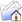LISTSERV Archives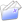CODE4LIB HomeCODE4LIB October 2013

Subject:Re: solr computation field norm problem

From:Date:Tue, 1 Oct 2013 12:24:08 +0200

Content-Type:text/plain

Parts/Attachments: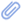text/plain (243 lines)
 ```Thanks, but the "sweet spot" in our metadata cannot be derived from its length. I'm rather searching for a similarity class that uses more than one byte to encode its field norm. I keep on digging ;-) ________________________________________ From: Code for Libraries [[log in to unmask]] On Behalf Of Erik Hatcher [[log in to unmask]] Sent: Thursday, September 26, 2013 3:07 PM To: [log in to unmask] Subject: Re: [CODE4LIB] solr computation field norm problem Nicolas - Lucene 4 still encodes norms, as described here: using this function: You might want to give SweetSpotSimilarity a try:         Erik On Sep 26, 2013, at 8:02 AM, Nicolas Franck <[log in to unmask]> wrote: > I've been testing with Solr 4 (Lucene 4) that uses the new DefaultSimilarity class. > It does not use the "encodeNorm" and "decodeNorm" methods anymore that > caused all the trouble (storing the floats as a single byte). But it doesn't change anything? > The field norms remain the same? > ________________________________________ > From: Code for Libraries [[log in to unmask]] On Behalf Of Chris Fitzpatrick [[log in to unmask]] > Sent: Wednesday, September 25, 2013 7:57 PM > To: [log in to unmask] > Subject: Re: [CODE4LIB] solr computation field norm problem > > Yeah...I think you're running into this: > > http://lucene.472066.n3.nabble.com/field-length-normalization-tp495308p495311.html > > TL;DR: > Jay Hill says fields with 3 terms and 4 terms both score at .5 in the > lengthNorm. > > > > > > > > On Wed, Sep 25, 2013 at 4:21 PM, Nicolas Franck <[log in to unmask]>wrote: > >> Hi there, >> >> I have a question about the way Lucene computes the length norm of field >> norm for its documents. >> My documents are indexed using Solr. >> These are the documents that where indexed (ignore 'score', that is not >> part of the document itself) >> >> >> 1.00711 >> ejn01:2560000000075596 >> Journal of neurology research >> >> >> 1.00711 >> ejn01:954925518616 >> Journal of neurology >> >> >> >> The field "title" has the following definition in schema.xml: >> >> > positionIncrementGap="100" omitNorms="false"> >> >> > maxTokenLength="1024"/> >> >> >> > synonyms="index_synonyms.txt" format="solr" ignoreCase="false" >> expand="true" tokenizerFactory="solr.WhitespaceTokenizerFactory"/> >> >> >> > maxTokenLength="1024"/> >> >> >> > synonyms="index_synonyms.txt" format="solr" ignoreCase="false" >> expand="true" tokenizerFactory="solr.WhitespaceTokenizerFactory"/> >> >> >> >> >> If I use the query "journal of neurology", both documents have the same >> score, although the second document is more exact. Supplying a phrase query >> does not fix the issue. I also see that the computed fieldNorm is "0.5" for >> both documents. Does this have something to do with the loss of precision >> when storing the length norm into one byte? >> >> These are all the supplied parameters (defaults in solrconfig.xml): >> >> false >> -10% >> author^3 title^2 >> score desc >> >> source:ser01^10 >> source:ejn01^10 >> (*:* -type:article)^999 >> >> all >> all >> 0 >> >> author^15 title^10 subject^1 summary^1 library^1 location^1 publisher^1 >> place_published^1 issn^1 isbn^1 >> >> *:* >> 2 >> edismax >> journal of neurology >> all >> score desc >> >> Looking the computation of the score, I see no single difference between >> them (see down below) >> Any idea why the fieldNorm is the same for both documents? >> >> >> Thanks in advance! >> >> Greetings, >> >> Nicolas >> >> >> >> >> >> 1.0071099 = (MATCH) sum of: >> 0.0053001107 = (MATCH) sum of: >> 0.0017667036 = (MATCH) max of: >> 0.0017667036 = (MATCH) weight(title:journal^10.0 in 0), product of: >> 0.005943145 = queryWeight(title:journal^10.0), product of: >> 10.0 = boost >> 0.5945349 = idf(docFreq=2, maxDocs=2) >> 9.996294E-4 = queryNorm >> 0.29726744 = (MATCH) fieldWeight(title:journal in 0), product of: >> 1.0 = tf(termFreq(title:journal)=1) >> 0.5945349 = idf(docFreq=2, maxDocs=2) >> 0.5 = fieldNorm(field=title, doc=0) >> 0.0017667036 = (MATCH) max of: >> 0.0017667036 = (MATCH) weight(title:of^10.0 in 0), product of: >> 0.005943145 = queryWeight(title:of^10.0), product of: >> 10.0 = boost >> 0.5945349 = idf(docFreq=2, maxDocs=2) >> 9.996294E-4 = queryNorm >> 0.29726744 = (MATCH) fieldWeight(title:of in 0), product of: >> 1.0 = tf(termFreq(title:of)=1) >> 0.5945349 = idf(docFreq=2, maxDocs=2) >> 0.5 = fieldNorm(field=title, doc=0) >> 0.0017667036 = (MATCH) max of: >> 0.0017667036 = (MATCH) weight(title:neurology^10.0 in 0), product of: >> 0.005943145 = queryWeight(title:neurology^10.0), product of: >> 10.0 = boost >> 0.5945349 = idf(docFreq=2, maxDocs=2) >> 9.996294E-4 = queryNorm >> 0.29726744 = (MATCH) fieldWeight(title:neurology in 0), product of: >> 1.0 = tf(termFreq(title:neurology)=1) >> 0.5945349 = idf(docFreq=2, maxDocs=2) >> 0.5 = fieldNorm(field=title, doc=0) >> 0.0031800664 = (MATCH) max of: >> 0.0031800664 = (MATCH) weight(title:"journal of neurology"~2^2.0 in >> 0), product of: >> 0.0035658872 = queryWeight(title:"journal of neurology"~2^2.0), >> product of: >> 2.0 = boost >> 1.7836046 = idf(title: journal=2 of=2 neurology=2) >> 9.996294E-4 = queryNorm >> 0.8918023 = fieldWeight(title:"journal of neurology" in 0), product >> of: >> 1.0 = tf(phraseFreq=1.0) >> 1.7836046 = idf(title: journal=2 of=2 neurology=2) >> 0.5 = fieldNorm(field=title, doc=0) >> 0.99862975 = (MATCH) sum of: >> 0.99862975 = (MATCH) MatchAllDocsQuery, product of: >> 0.99862975 = queryNorm >> >> >> 1.0071099 = (MATCH) sum of: >> 0.0053001107 = (MATCH) sum of: >> 0.0017667036 = (MATCH) max of: >> 0.0017667036 = (MATCH) weight(title:journal^10.0 in 1), product of: >> 0.005943145 = queryWeight(title:journal^10.0), product of: >> 10.0 = boost >> 0.5945349 = idf(docFreq=2, maxDocs=2) >> 9.996294E-4 = queryNorm >> 0.29726744 = (MATCH) fieldWeight(title:journal in 1), product of: >> 1.0 = tf(termFreq(title:journal)=1) >> 0.5945349 = idf(docFreq=2, maxDocs=2) >> 0.5 = fieldNorm(field=title, doc=1) >> 0.0017667036 = (MATCH) max of: >> 0.0017667036 = (MATCH) weight(title:of^10.0 in 1), product of: >> 0.005943145 = queryWeight(title:of^10.0), product of: >> 10.0 = boost >> 0.5945349 = idf(docFreq=2, maxDocs=2) >> 9.996294E-4 = queryNorm >> 0.29726744 = (MATCH) fieldWeight(title:of in 1), product of: >> 1.0 = tf(termFreq(title:of)=1) >> 0.5945349 = idf(docFreq=2, maxDocs=2) >> 0.5 = fieldNorm(field=title, doc=1) >> 0.0017667036 = (MATCH) max of: >> 0.0017667036 = (MATCH) weight(title:neurology^10.0 in 1), product of: >> 0.005943145 = queryWeight(title:neurology^10.0), product of: >> 10.0 = boost >> 0.5945349 = idf(docFreq=2, maxDocs=2) >> 9.996294E-4 = queryNorm >> 0.29726744 = (MATCH) fieldWeight(title:neurology in 1), product of: >> 1.0 = tf(termFreq(title:neurology)=1) >> 0.5945349 = idf(docFreq=2, maxDocs=2) >> 0.5 = fieldNorm(field=title, doc=1) >> 0.0031800664 = (MATCH) max of: >> 0.0031800664 = (MATCH) weight(title:"journal of neurology"~2^2.0 in >> 1), product of: >> 0.0035658872 = queryWeight(title:"journal of neurology"~2^2.0), >> product of: >> 2.0 = boost >> 1.7836046 = idf(title: journal=2 of=2 neurology=2) >> 9.996294E-4 = queryNorm >> 0.8918023 = fieldWeight(title:"journal of neurology" in 1), product >> of: >> 1.0 = tf(phraseFreq=1.0) >> 1.7836046 = idf(title: journal=2 of=2 neurology=2) >> 0.5 = fieldNorm(field=title, doc=1) >> 0.99862975 = (MATCH) sum of: >> 0.99862975 = (MATCH) MatchAllDocsQuery, product of: >> 0.99862975 = queryNorm >> >>```

#### Search Archives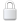Log In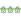Get Password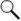Search Archives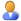Subscribe or Unsubscribe